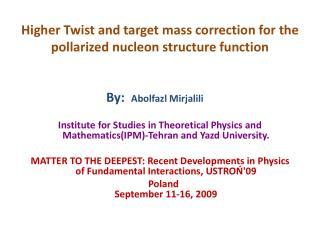DownloadDownload PresentationHigher Twist and target mass correction for the pollarized nucleon structure function

# Higher Twist and target mass correction for the pollarized nucleon structure function

Télécharger la présentation## Higher Twist and target mass correction for the pollarized nucleon structure function

- - - - - - - - - - - - - - - - - - - - - - - - - - - E N D - - - - - - - - - - - - - - - - - - - - - - - - - - -
##### Presentation Transcript

1. Higher Twist and target mass correction for the pollarized nucleon structure function By: Abolfazl Mirjalili Institute for Studies in Theoretical Physics and Mathematics(IPM)-Tehran and Yazd University. MATTER TO THE DEEPEST: Recent Developments in Physics of Fundamental Interactions, USTROŃ'09 PolandSeptember 11-16, 2009

2. Contenents: *Review on Particles Physics * Structure functions * Constiuent Quark model * Polarized Structure function of Poton * Mass effect Correction

3. Review on Particles Physics Schrodinger Eq. Kelein_Gordon Eq. Dirac Eq.

4. Review on Particles Physics • Quantum Electro • Dynamic Quantum Field Theory • Quantum Chromo Dynamic Quark-Parton Model

5. Review on Particles Physics Bjrorken Scaling Violation of Bjorken Scaling Eqs.DGLAP

6. Structure function Un-polarized structure function

7. Structure function Scattering X- section

8. Structure function Polarized SF

9. Constituent Quark Model Valon Model SF in V-Model;Convolution Int V-distribution F inside the P

10. Constituent Quark Model • Moment space * Mellein Transformation *

11. Constituent Quark Model • Distribution function in Moment space*

12. Constituent Quark Model • Relation between polarized and un-polarized V-distribution For Non-Singlet Sector* For Singlet Sector*

13. Proton polarized SF: g1(x,Q2) at Leading Order approximationg1(x,Q2)

14. Proton polarized SF:g1(x,Q2) Evolution of Non-Singlet distribution of active flavor with Q^2 • Evolution of Singlet distribution of active flavor and gluon distribution with Q^2

15. Proton polarized SF:g1(x,Q2) • Evolution of distribution in moment space

16. Proton polarized SF: g1(x,Q2) Evolution of Non-singlet distribution with Q^2 in LO approximation • Evolution of singlet and gluon distribution with Q^2 in LO approximation

17. Proton polarized SF:g1(x,Q2) Parton distribution function inside the proton

18. Proton polarized SF: g1(x,Q2) Proton polarized SF in moment space and in LO approximation

19. Proton polarized SF: g1(x,Q2) Fitting and Jaccobi polynomials

20. Proton polarized SF:g1(x,Q2) Comparison of proton polarized SF with experimental data using V-model in LO approximation and at Q^2= 2 Gev^2

21. Proton polarized SF:g1(x,Q2) SF in NLO approximation and in moment spaceg1(x,Q2)

22. Proton polarized SF:g1(x,Q2) Comparison of proton polarized SF with experimental data using V-model in NLO approximation and at Q^2= 2 Gev^2

23. Mass Effect Correction Natchman variable:

24. Mass Effect Correction • Mass effect relation for g1(x,Q2) in terms of first and third twist operator matrix element till third order of mass approximatio

25. Mass Effect Correction

26. Mass Effect Correction • Comparison of proton polarized SF ,g1(x,Q2), with experimental data using V-model in LO approximation and at Q^2= 2 Gev^2. The mass effect result is also included.

27. Mass Effect Correction • Comparison of proton polarized SF ,g1(x,Q2), with experimental data using V-model in LO approximation and at Q^2= 2 Gev^2. The mass effect result is also included.